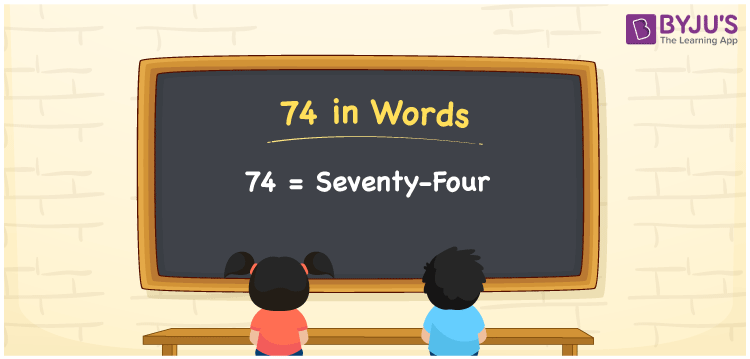# 74 in Words

74 in words is written as Seventy-four. In both the International and the Indian System of Numerals, 74 in words is written as Seventy-four. The number 74 is a cardinal number as 74 can represent some quantity. For example, “74 bananas are there in the basket” here, 74 represents the number of bananas.

 74 in Words Seventy-four Seventy-four in Numbers 74

## 74 in English Words

We write 74 in English Words using the letters of English alphabets. Therefore, we spell 74 in English as “Seventy-four.”## How to Write 74 in Words?

To write 74 in words, we shall use the place value chart. In the place value chart, write 4 in the ones place and 7 in the tens place. Let us make a place value chart to write the number 74 in words.

 Tens Ones 7 4

Thus, we can write the expanded form as

7 × Ten + 4 × One

= 7 × 10 + 4 × 1

= 70 + 4

= 74

= Seventy-four.

74 is the 74th natural number which is the successor of 73 and the predecessor of 75.

74 in words – Seventy-four

• Is 74 an odd number? – No
• Is 74 an even number? – Yes
• Is 74 a perfect square number? – No
• Is 74 a perfect cube number? – No
• Is 74 a prime number? – No
• Is 74 a composite number? – Yes

## Frequently Asked Questions on 74 in Words

Q1

### How to write 74 in English words?

74 in words is written as Seventy-four.
Q2

### How to spell 74?

The spelling of 74 is “Seventy-four.”
Q3

### Is 74 a Whole Number?

Yes, 74 is a Whole Number.